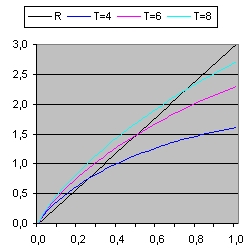### Milne's model Universe

For a very good introduction read this: Introducing the Dirac-Milne universe by Aurélien Benoit-Lévy, Gabriel Chardin Februari 2012

The purpose of this document is to demonstrate the evolution of the universe when space expansion is linear. This is called: Milne's model.
The differential equation for this type of universe is: dR/dt = c

The solution is R = c*t
In order to demonstrate also an EXCEL program is available. In order to download this program select: linear expansion.xls.zip

For a general introduction about World Models read this: 3 World models

### Program Operation

The Excel program "linear expansion.xls" contains of one "page". This page contains two parameters c and type and one command: Calculate
• The parameter c defines the speed of the outer edge of the universe. When c=1 this is the speed of light. Two values are discussed C=1 and c=2.
• The parameter type defines how the light path is calculated.
• When type = 1 the starting point is a point at the outer edge of the universe. The light curve shows the path starting from this event until it reaches the observer.
• When type = 2 the light curve is calculated (specific the starting point) such that it reaches the observer at a fixed moment.
• The Command Calculate is used to perform one simulation.
At each simulation three light curves are calculated.
When the radius at iteration n is R(n) and the distance of the lightray is dist(n) than :
1. R(n+1) = R(n) + c * dt
2. dist(n+1) = dist(n) * R(n+1) / R(n) - dt
Equation 1 shows the new radius of the universe.
Equation 2 shows the new distance of the event. The first part shows space expansion and the second part shows the distance of the light towards the observer.

### ReflectionPicture 5 C = 3 Type = 2 Picture 5 shows the evalution of three light signals when the expansion speed = c = 3. The target times are 5, 10 and 15 billion years. Picture 5 only shows the lightcurve for the first billion years. What is important in none of these three cases you will be able to observe the Big Bang. In the case of the blue line (Target time is 5 billion years) the earliest events that can be observed happend roughly 250000 years after the Big Bang. To observe the Big Bang (galaxies very close to the Big Bang) the expansion speed should be much higher. To introduce a period of inflation helps.